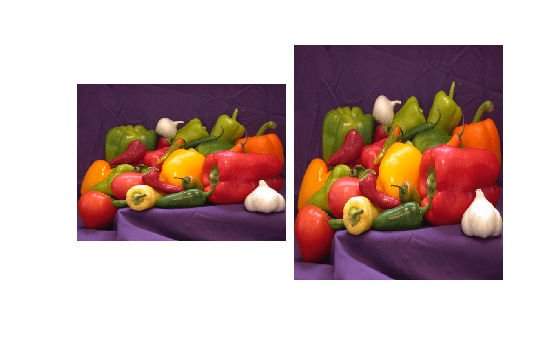# dlresize

Resize spatial dimensions of `dlarray` object

Since R2020b

## Syntax

``Y = dlresize(X,'Scale',scale)``
``Y = dlresize(X,'OutputSize',outputSize)``
``Y = dlresize(___,Name,Value)``

## Description

example

````Y = dlresize(X,'Scale',scale)` resizes the spatial dimensions of the `dlarray` object `X` by a scale factor `scale`.This function requires Deep Learning Toolbox™.```
````Y = dlresize(X,'OutputSize',outputSize)` resizes the spatial dimensions of the `dlarray` object `X` so that the spatial dimension sizes are equal to `outputSize`.```
````Y = dlresize(___,Name,Value)` adjusts the resizing operation using name-value pair arguments. If `X` is not a formatted `dlarray`, then you must specify the `DataFormat` name-value pair argument.```

## Examples

collapse all

`A = imread('peppers.png');`

Convert the image to data type `single` for use in a `dlarray`. Then, create a `dlarray` containing the input image.

```A = im2single(A); dlarrayA = dlarray(A,'SSC');```

Rescale the `dlarray` by a factor of 1.5 vertically.

`dlarrayB = dlresize(dlarrayA,'Scale',[1.5 1]);`

Extract the image data from the resized `dlarray` `B` by using the `extractdata` (Deep Learning Toolbox) function.

`B = extractdata(dlarrayB);`

Display the original and resized images as a montage.

```montage({A,B},"ThumbnailSize",size(B,[1 2]), ... "BorderSize",10,"BackgroundColor","white")```## Input Arguments

collapse all

Deep learning array to resize, specified as a `dlarray` (Deep Learning Toolbox) object.

Scale factor to resize input, specified as a positive number or a vector of positive numbers of length equal to the number of spatial dimensions in `X`. If `scale` is a scalar, then `dlresize` applies the same scale factor to all spatial dimensions.

Output size of resized input, specified as a vector of positive integers of length equal to the number of spatial dimensions in `X`. You can specify one element as a positive integer and specify the other elements as NaN, in which case the layer computes the other elements automatically to preserve the aspect ratio of the input.

### Name-Value Arguments

Specify optional pairs of arguments as `Name1=Value1,...,NameN=ValueN`, where `Name` is the argument name and `Value` is the corresponding value. Name-value arguments must appear after other arguments, but the order of the pairs does not matter.

Before R2021a, use commas to separate each name and value, and enclose `Name` in quotes.

Example: `'Method',linear`

Dimension labels of the input `dlarray` object `X`, specified as the comma-separated pair consisting of `'DataFormat'` and a string scalar or character vector. Each character must be one of these labels:

• `S` — Spatial

• `C` — Channel

• `B` — Batch observations

• `T` — Time or sequence

• `U` — Unspecified

If `X` is not a formatted `dlarray`, then you must specify the `DataFormat` name-value pair argument. For more information, see `dlarray` (Deep Learning Toolbox).

Example: `'SSC'` indicates the array has two spatial dimensions and one channel dimension, appropriate for 2-D RGB image data.

Interpolation method, specified as the comma-separated pair consisting of `'Method'` and `"nearest"` for nearest neighbor interpolation or `"linear"` for bilinear interpolation.

Geometric transformation mode to map points from input space to output space, specified as the comma-separated pair consisting of `'GeometricTransformMode'` and `"half-pixel"` or `"asymmetric"`.

Rounding mode for nearest neighbor interpolation, specified as the comma-separated pair consisting of `'NearestRoundingMode'` and one of the following.

• `"round"` — use the same rounding behavior as the MATLAB® `round` function.

• `"floor"` — use the same rounding behavior as the MATLAB `floor` function.

• `"onnx-10"` — reproduce the resizing behavior of the ONNX™ (Open Neural Network Exchange) opset 10 Resize operator.

This argument is used when you specify the `Method` as `'nearest'`.

## Output Arguments

collapse all

Resized deep learning array, returned as a `dlarray` (Deep Learning Toolbox) object.

## Version History

Introduced in R2020b